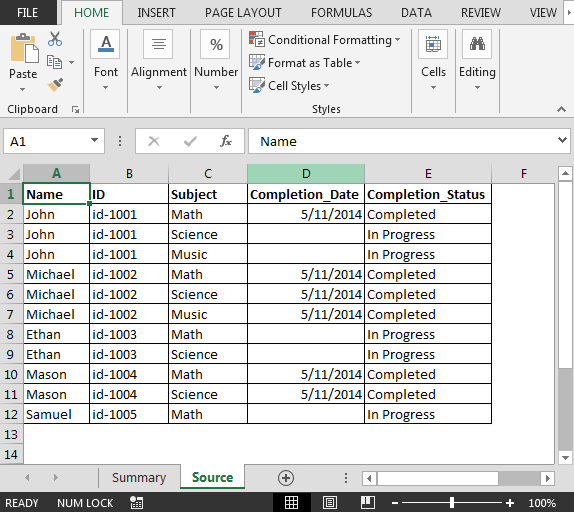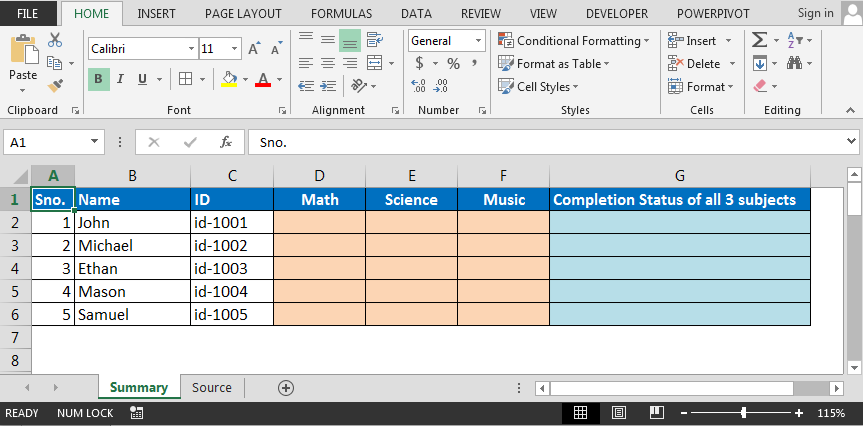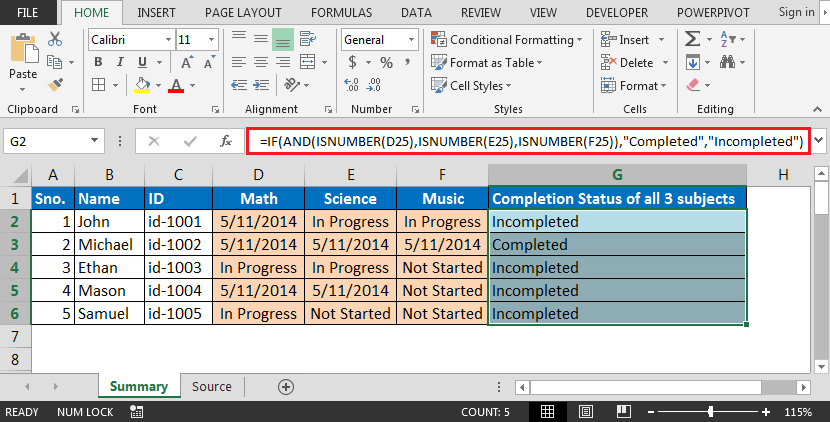# Extract Student Summary Data from Another Sheet Based on Various Status in Microsoft Excel

In this article, you will learn how to get summary status of student from Source sheet to Summary sheet based on multiple checks.

Question:-

I have two sheets i.e. Source & Summary. In Source sheet, we have Name of Student, ID, Subject, Completion Date & Completion Status.

• I want a formula to check for each student & subject in Source sheet & extract completion date if not completed then pull the status.

Following is the snapshot of Source sheet:Following is the snapshot of Summary sheet:• In cell D2, the formula is { =IFERROR(IF(INDEX(Source!\$D\$2:\$D\$12,MATCH(1,(Source!\$A\$2:\$A\$12=\$B2)*(Source!\$C\$2:\$C\$12=D\$1),0))=0,INDEX(Source!\$E\$2:\$E\$12,MATCH(1,(Source!\$A\$2:\$A\$12=\$B2)*(Source!\$C\$2:\$C\$12=D\$1),0)),INDEX(Source!\$D\$2:\$D\$12,MATCH(1,(Source!\$A\$2:\$A\$12=\$B2)*(Source!\$C\$2:\$C\$12=D\$1),0))),"Not Started")}

Note: This is an array formula; you are required to use CTRL + SHIFT + ENTER keys together

• Drag down & across the formula in range D2:F6; we will get the status of all three subjects:• To update the combined status of all three subjects for each student marks In column G, we will use formula as =IF(AND(ISNUMBER(D25),ISNUMBER(E25),ISNUMBER(F25)),"Completed","Incompleted")In this way, we can extract student summary data based on data in anther sheet.

1.